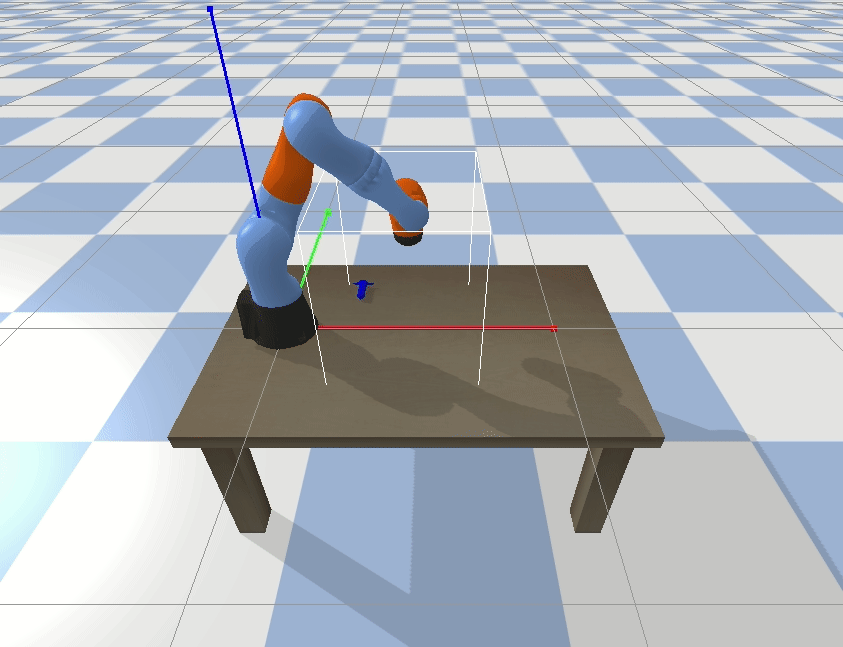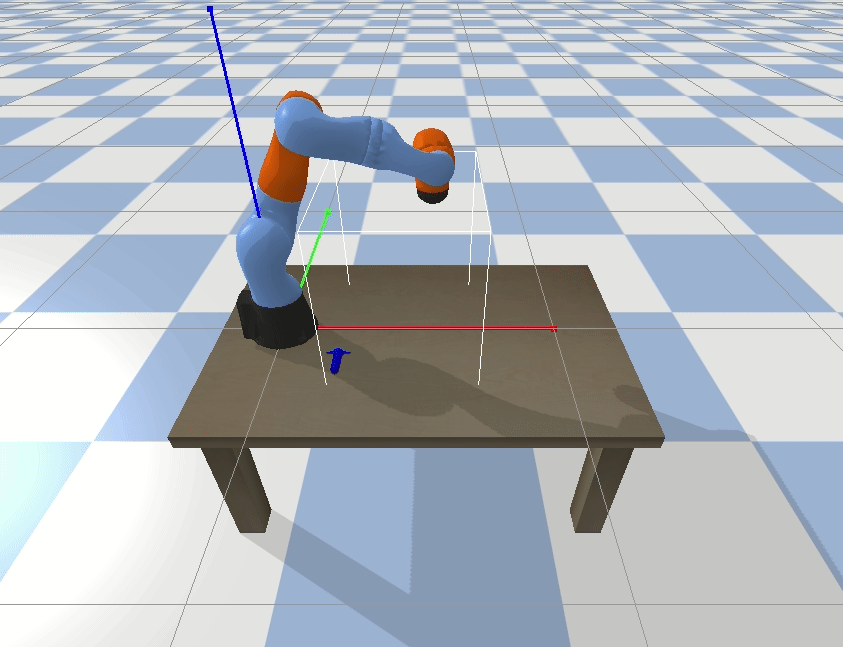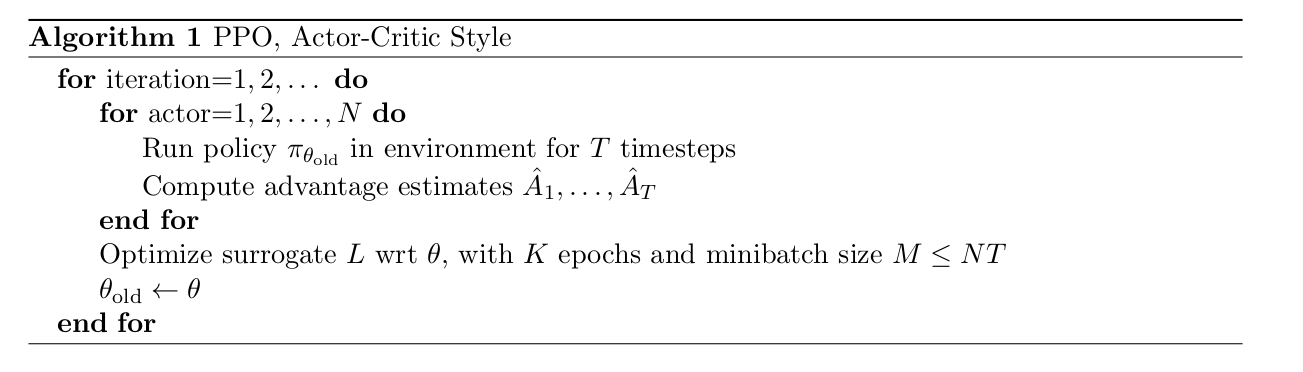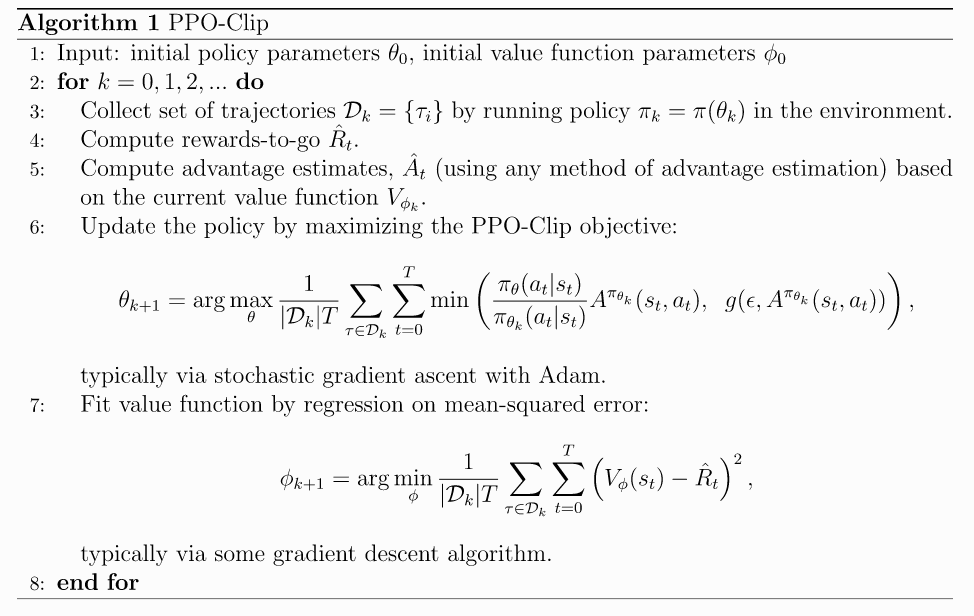• 算法主要包含收集经验数据
• 使用GAE来估计优势函数(Advantage Function)
• 设计value function和policy function
• 对于policy，使用PPO-clip更新policy的参数
• 更新value function的参数

# 整体思路

actor代表产生行为的函数，也就是Policy。critic代表评价行为的函数，也就是value function。actor需要根据当前的observation，来产生act，critic需要根据当前的observation，来评价当前的state的价值，而这个state是通过actor产生act之后，环境执行step得来的，所以这样critic就与actor联系了起来，critic来评价actor产生的行为的好坏。

• 如果想让机械臂正确的学会reach到一个点，基本的设计思路有以下几种：
（1）state为当前要reach的点的坐标，actor可以根据这个点的坐标决定自己的动作，critic可以根据这个点的坐标输出价值函数的值（这个地方重点看一下，有待商榷）；
（2）state为当前要reach点和机械臂末端末端两者的坐标，actor可以根据当前末端坐标和reach坐标输出动作，critic也可以更容易的根据当前末端坐标和reach坐标生成价值的判断；
（3）state为当前工作场景的图像信息，actor根据当前的图像生成动作，critic根据当前的图像做出判断，这个是最难得，不过最后我们也会实现。

• 那么有些小伙伴会说，除了第三种方法，前两种方法我直接机械臂求逆解过去不就是嘛。事情确实是这么个事情，道理也是这样的道理，只是在这里我们强行强化学习了。
• 我们先从思路（1）开始，此时的state为reach点的坐标，因为是完全MDP问题，所以observation=state，此时的observation=[reach_x, reach_y, reach_z]， 是一个1x3的向量。

• 确定了observation之后，紧接着是怎样设计动作，也就是让actor输出什么？ 是用离散的动作输出还是连续的动作输出？ 输出关节角度？ 输出末端坐标？ 还是定义离散方向，让机械臂按照方向运动？ 大家可以停下来思考一下。
• 我在这里采用的是输出末端坐标。因为有了末端坐标，可以直接控制机械臂到达这个坐标点。因为输出的坐标值，所以这是一个连续的输出控制问题。
• 现在确定了observation，也就是actor和critic的输入，也确定了actor的输出，critic的输出是固定的，就是一个数值，来表示当前的价值。所以我们就可以编写actor和critic的函数了。

# critic

actor的工作流程就是
observation(1x3) -> critic -> obs value(1x1)

import torch
import torch.nn as nn

def mlp(sizes, activation, output_activation=nn.Identity):
layers = []
for j in range(len(sizes) - 1):
act = activation if j < len(sizes) - 2 else output_activation
layers += [nn.Linear(sizes[j], sizes[j + 1]), act()]
return nn.Sequential(*layers)


mlp的编写很简单，逻辑也很清楚，可以通过

obs_dim=3
hidden_sizes=[64,64]
activation=nn.Tanh

net=mlp([obs_dim] + list(hidden_sizes) + , activation)
print(net)


class MLPCritic(nn.Module):

def __init__(self, obs_dim, hidden_sizes, activation):
super().__init__()
self.v_net = mlp([obs_dim] + list(hidden_sizes) + , activation)

def forward(self, obs):
return torch.squeeze(self.v_net(obs), -1)  # Critical to ensure v has right shape.


import torch
import torch.nn as nn

def mlp(sizes, activation, output_activation=nn.Identity):
layers = []
for j in range(len(sizes) - 1):
act = activation if j < len(sizes) - 2 else output_activation
layers += [nn.Linear(sizes[j], sizes[j + 1]), act()]
return nn.Sequential(*layers)

class MLPCritic(nn.Module):

def __init__(self, obs_dim, hidden_sizes, activation):
super().__init__()
self.v_net = mlp([obs_dim] + list(hidden_sizes) + , activation)

def forward(self, obs):
return torch.squeeze(self.v_net(obs), -1)  # Critical to ensure v has right shape.

obs_dim=3
observation=torch.as_tensor([0.5, 0.1, 0],dtype=torch.float32)
hidden_sizes=[64,64]
activation=nn.Tanh

critic=MLPCritic(obs_dim,hidden_sizes,activation)
print('v_net={}'.format(critic.v_net))
print('v_net(obs)={}'.format(critic.v_net(observation)))
print('v_net forward={}'.format(critic.forward(observation)))


>

v_net=Sequential(
(0): Linear(in_features=3, out_features=64, bias=True)
(1): Tanh()
(2): Linear(in_features=64, out_features=64, bias=True)
(3): Tanh()
(4): Linear(in_features=64, out_features=1, bias=True)
(5): Identity()
)
v_net(obs)=tensor([0.1236], grad_fn=)
v_net forward=0.12357600778341293